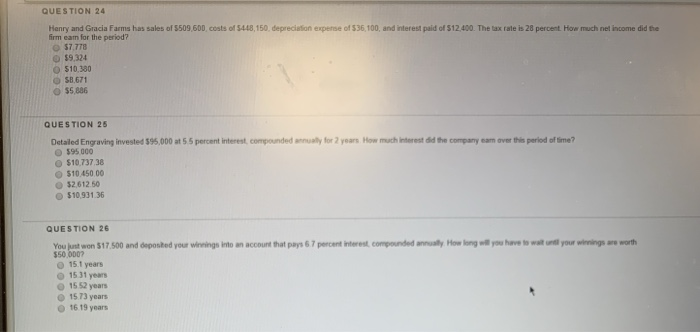Question

QUESTION 24 Henry and Gracia Farms has sales of 5509,60D, costs of 5448,150, depreciation expense of...QUESTION 24 Henry and Gracia Farms has sales of 5509,60D, costs of 5448,150, depreciation expense of 536,100, and interest paid of 512,400. The tax rate is 28 percent. How much net income did the firm eam for the period? 57.778 \$9324 \$10.280 S01 671 55,886 55 percent interest, compounded all for years. How much interested the company came s period of time? QUESTION 25 Detailed Engraving invested 195.000 595 000 510 737 38 \$10.50 00 32612 50 \$10.931 36 QUESTION 26 You just won 517.500 and deposted your winning into an account that pays 67 percent interest compounded annually. How long will you have to wait until your winnings are worth \$50 000? 151 years 15 31 years 15 52 years 15 73 years 16 19 years

Taxable Income = Sales - Costs - Depreciation Expense - Interest Expense
Taxable Income = \$509,600 - \$448,150 - \$36,100 - \$12,400
Taxable Income = \$12,950

Net Income = Taxable Income * (1 - Tax Rate)
Net Income = \$12,950 * (1 - 0.28)
Net Income = \$9,324

Future Value = Amount Invested * (1 + Interest Rate)^Period
Future Value = \$95,000 * 1.055^2
Future Value = \$105,737.38

Interest Earned = Future Value - Amount Invested
Interest Earned = \$105,737.38 - \$95,000.00
Interest Earned = \$10,737.38

Amount Invested * (1 + Interest Rate)^Period = Future Value
\$17,500 * 1.067^Period = \$50,000
1.067^Period = 2.857143
Period * ln(1.067) = ln(2.857143)
Period = 16.19 years

Earn Coins

Coins can be redeemed for fabulous gifts.

Similar Homework Help Questions